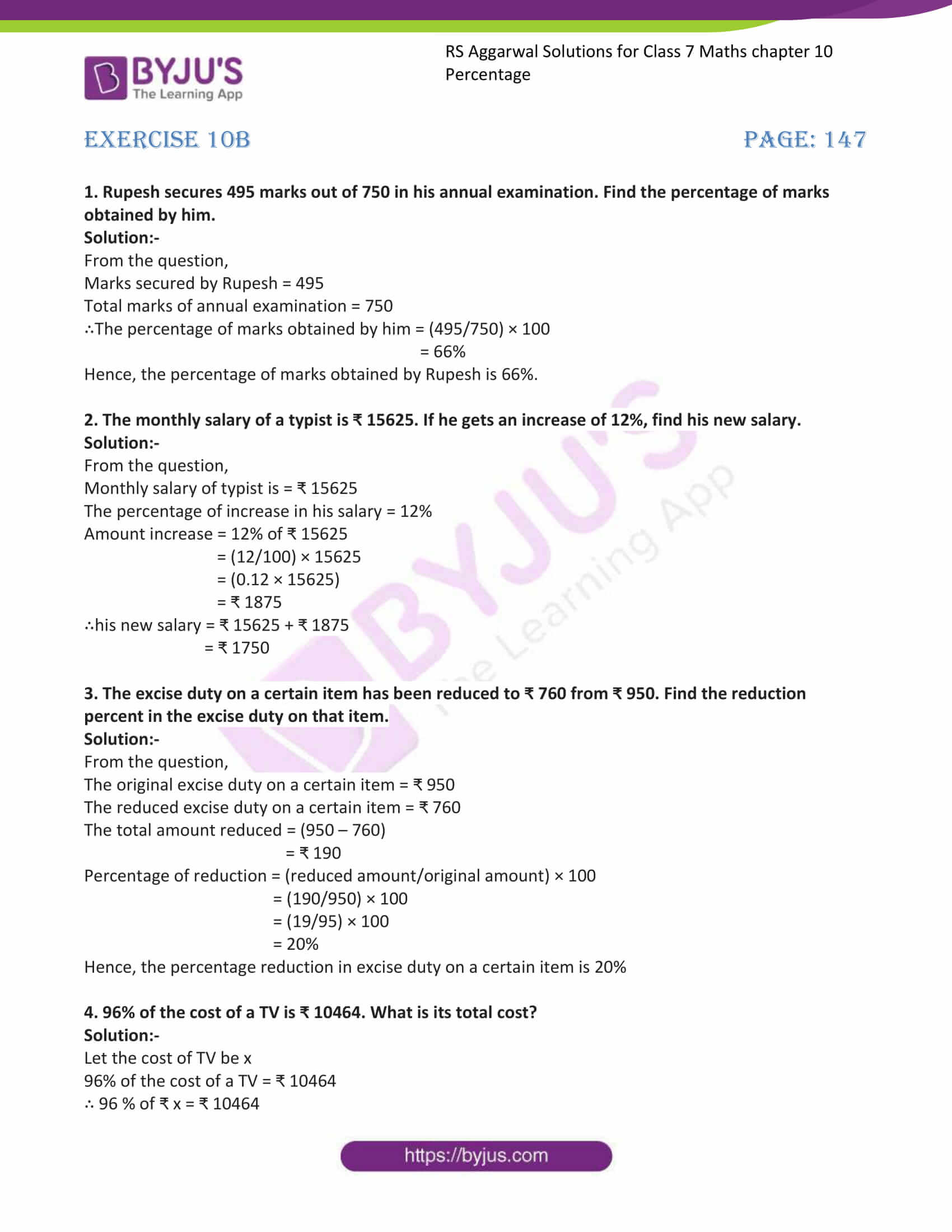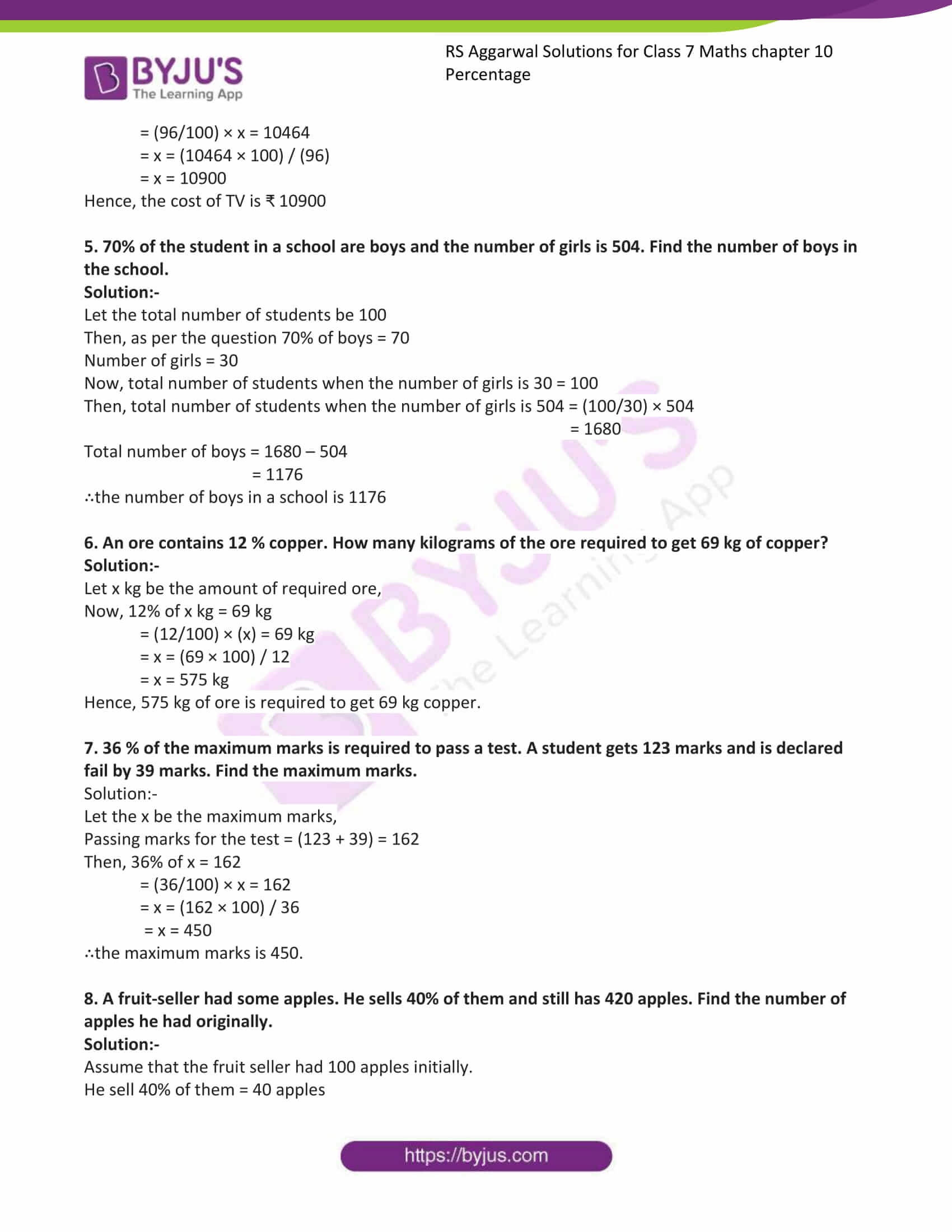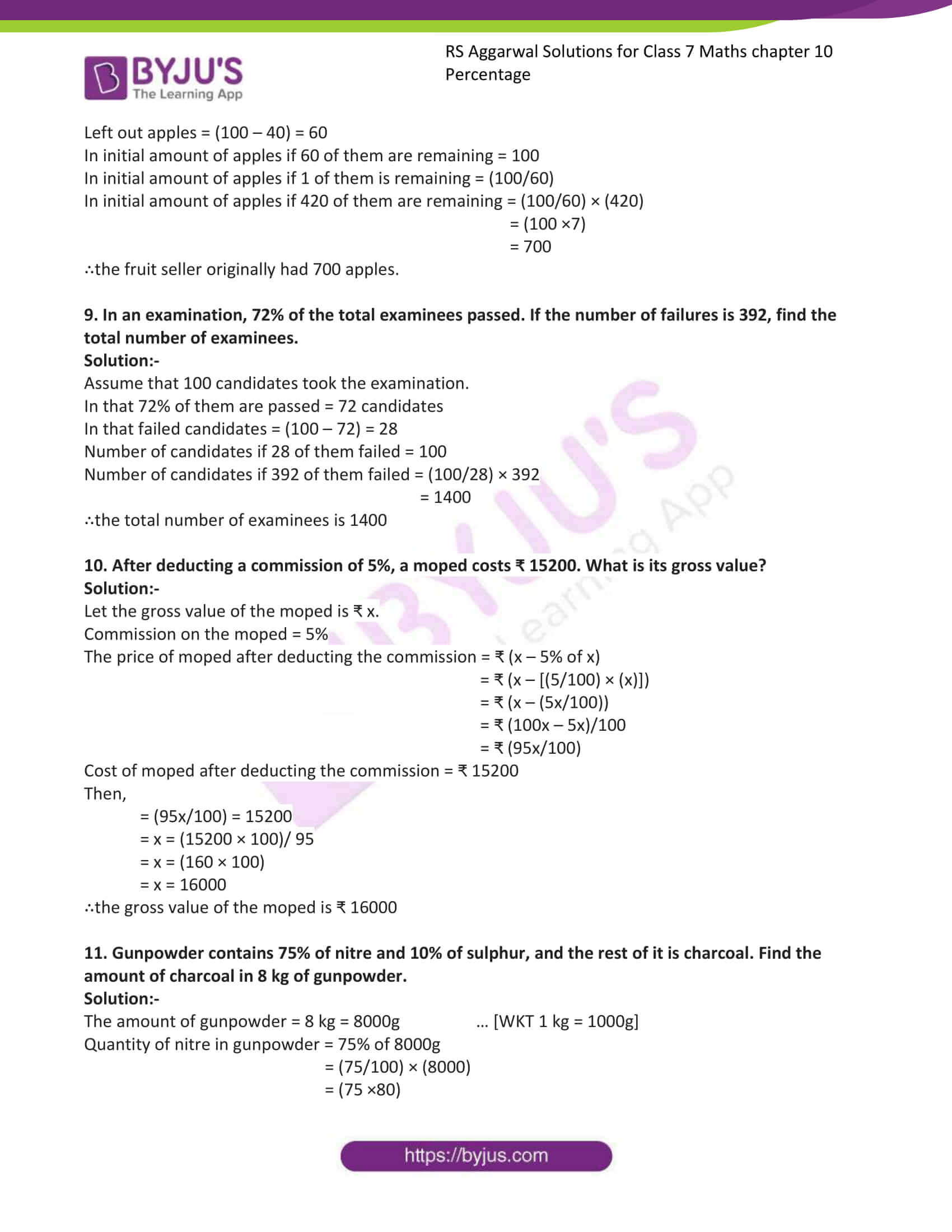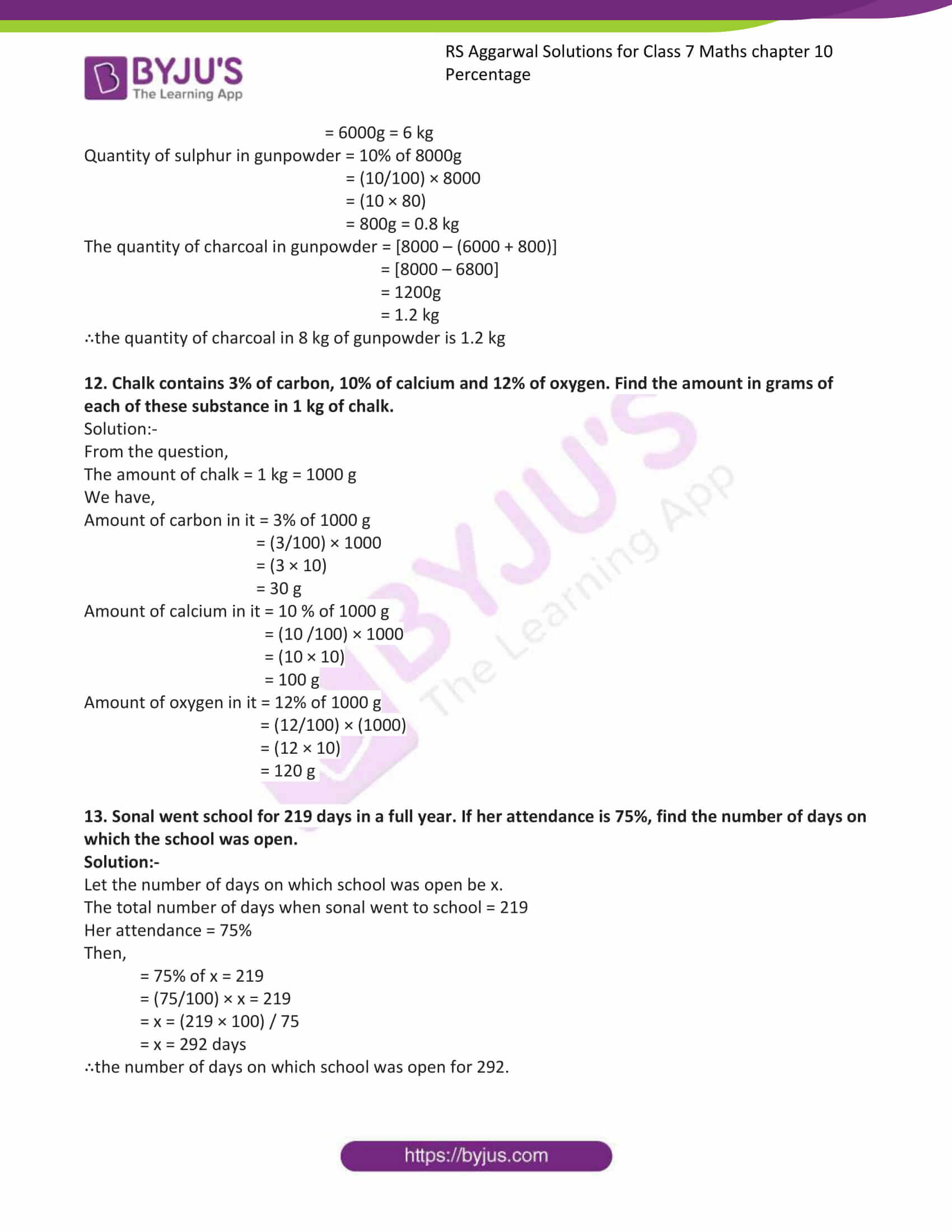# RS Aggarwal Solutions for Class 7 Maths Exercise 10B Chapter 10 Percentage

RS Aggarwal Solutions for Exercise 10B of Class 7 Maths Chapter 10 Percentage, this exercise is entirely based on word problems. By solving these word problems, students can improve their sentence formation. We at BYJU’S have prepared the RS Aggarwal Solutions for Class 7 Maths Chapter 10 Percentage wherein problems are solved step by step with detailed explanations. To cover the entire syllabus in Maths, the RS Aggarwal Solutions For Class 7 is a necessary material as it gives a wide range of questions that test the students’ understanding of concepts.

## Download the PDF of RS Aggarwal Solutions For Class 7 Maths Chapter 10 Percentage – Exercise 10B### Access answers to Maths RS Aggarwal Solutions for Class 7 Chapter 10 – Percentage Exercise 10B

1. Rupesh secures 495 marks out of 750 in his annual examination. Find the percentage of marks obtained by him.

Solution:-

From the question,

Marks secured by Rupesh = 495

Total marks of annual examination = 750

∴The percentage of marks obtained by him = (495/750) × 100

= 66%

Hence, the percentage of marks obtained by Rupesh is 66%.

2. The monthly salary of a typist is ₹ 15625. If he gets an increase of 12%, find his new salary.

Solution:-

From the question,

Monthly salary of typist is = ₹ 15625

The percentage of increase in his salary = 12%

Amount increase = 12% of ₹ 15625

= (12/100) × 15625

= (0.12 × 15625)

= ₹ 1875

∴his new salary = ₹ 15625 + ₹ 1875

= ₹ 1750

3. The excise duty on a certain item has been reduced to ₹ 760 from ₹ 950. Find the reduction percent in the excise duty on that item.

Solution:-

From the question,

The original excise duty on a certain item = ₹ 950

The reduced excise duty on a certain item = ₹ 760

The total amount reduced = (950 – 760)

= ₹ 190

Percentage of reduction = (reduced amount/original amount) × 100

= (190/950) × 100

= (19/95) × 100

= 20%

Hence, the percentage reduction in excise duty on a certain item is 20%

4. 96% of the cost of a TV is ₹ 10464. What is its total cost?

Solution:-

Let the cost of TV be x

96% of the cost of a TV = ₹ 10464

∴ 96 % of ₹ x = ₹ 10464

= (96/100) × x = 10464

= x = (10464 × 100) / (96)

= x = 10900

Hence, the cost of TV is ₹ 10900

5. 70% of the student in a school are boys and the number of girls is 504. Find the number of boys in the school.

Solution:-

Let the total number of students be 100

Then, as per the question 70% of boys = 70

Number of girls = 30

Now, total number of students when the number of girls is 30 = 100

Then, total number of students when the number of girls is 504 = (100/30) × 504

= 1680

Total number of boys = 1680 – 504

= 1176

∴the number of boys in a school is 1176

6. An ore contains 12 % copper. How many kilograms of the ore required to get 69 kg of copper?

Solution:-

Let x kg be the amount of required ore,

Now, 12% of x kg = 69 kg

= (12/100) × (x) = 69 kg

= x = (69 × 100) / 12

= x = 575 kg

Hence, 575 kg of ore is required to get 69 kg copper.

7. 36 % of the maximum marks is required to pass a test. A student gets 123 marks and is declared fail by 39 marks. Find the maximum marks.

Solution:-

Let the x be the maximum marks,

Passing marks for the test = (123 + 39) = 162

Then, 36% of x = 162

= (36/100) × x = 162

= x = (162 × 100) / 36

= x = 450

∴the maximum marks is 450.

8. A fruit-seller had some apples. He sells 40% of them and still has 420 apples. Find the number of apples he had originally.

Solution:-

Assume that the fruit seller had 100 apples initially.

He sell 40% of them = 40 apples

Left out apples = (100 – 40) = 60

In initial amount of apples if 60 of them are remaining = 100

In initial amount of apples if 1 of them is remaining = (100/60)

In initial amount of apples if 420 of them are remaining = (100/60) × (420)

= (100 ×7)

= 700

∴the fruit seller originally had 700 apples.

9. In an examination, 72% of the total examinees passed. If the number of failures is 392, find the total number of examinees.

Solution:-

Assume that 100 candidates took the examination.

In that 72% of them are passed = 72 candidates

In that failed candidates = (100 – 72) = 28

Number of candidates if 28 of them failed = 100

Number of candidates if 392 of them failed = (100/28) × 392

= 1400

∴the total number of examinees is 1400

10. After deducting a commission of 5%, a moped costs ₹ 15200. What is its gross value?

Solution:-

Let the gross value of the moped is ₹ x.

Commission on the moped = 5%

The price of moped after deducting the commission = ₹ (x – 5% of x)

= ₹ (x – [(5/100) × (x)])

= ₹ (x – (5x/100))

= ₹ (100x – 5x)/100

= ₹ (95x/100)

Cost of moped after deducting the commission = ₹ 15200

Then,

= (95x/100) = 15200

= x = (15200 × 100)/ 95

= x = (160 × 100)

= x = 16000

∴the gross value of the moped is ₹ 16000

11. Gunpowder contains 75% of nitre and 10% of sulphur, and the rest of it is charcoal. Find the amount of charcoal in 8 kg of gunpowder.

Solution:-

The amount of gunpowder = 8 kg = 8000g … [WKT 1 kg = 1000g]

Quantity of nitre in gunpowder = 75% of 8000g

= (75/100) × (8000)

= (75 ×80)

= 6000g = 6 kg

Quantity of sulphur in gunpowder = 10% of 8000g

= (10/100) × 8000

= (10 × 80)

= 800g = 0.8 kg

The quantity of charcoal in gunpowder = [8000 – (6000 + 800)]

= [8000 – 6800]

= 1200g

= 1.2 kg

∴the quantity of charcoal in 8 kg of gunpowder is 1.2 kg

12. Chalk contains 3% of carbon, 10% of calcium and 12% of oxygen. Find the amount in grams of each of these substance in 1 kg of chalk.

Solution:-

From the question,

The amount of chalk = 1 kg = 1000 g

We have,

Amount of carbon in it = 3% of 1000 g

= (3/100) × 1000

= (3 × 10)

= 30 g

Amount of calcium in it = 10 % of 1000 g

= (10 /100) × 1000

= (10 × 10)

= 100 g

Amount of oxygen in it = 12% of 1000 g

= (12/100) × (1000)

= (12 × 10)

= 120 g

13. Sonal went school for 219 days in a full year. If her attendance is 75%, find the number of days on which the school was open.

Solution:-

Let the number of days on which school was open be x.

The total number of days when sonal went to school = 219

Her attendance = 75%

Then,

= 75% of x = 219

= (75/100) × x = 219

= x = (219 × 100) / 75

= x = 292 days

∴the number of days on which school was open for 292.

### Access other exercises of RS Aggarwal Solutions For Class 7 Chapter 10 – Percentage

Exercise 10A Solutions

Exercise 10C Solutions